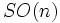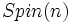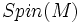# Spin structure

(Redirected from Spin manifold)
A spin structure on an oriented Riemannian manifold is a lift of its gluing cocycle to$SO(n)$, to a gluing cocycle to$Spin(n)$, the double cover of$SO(n)$.
The set of isomorphism classes of spin structures on a Riemannian manifold$M$ is denoted as$Spin(M)$.